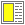Linguistic abstraction - slide 21 : 22

Scheme in Scheme
It is possible to write a relatively full, but brief meta circular Scheme interpreter in Scheme
```(define (eval exp env)
(cond ((self-evaluating? exp) exp)
((quoted? exp) (text-of-quotation exp))
((variable? exp) (lookup-variable-value exp env))
((definition? exp) (eval-definition exp env))
((assignment? exp) (eval-assignment exp env))
((lambda? exp) (make-procedure exp env))
((conditional? exp) (eval-cond (clauses exp) env))
((application? exp)  (apply (eval (operator exp) env)
(list-of-values (operands exp) env)))
(else (error "Unknown expression type -- EVAL" exp))))

(define (apply procedure arguments)
(cond ((primitive-procedure? procedure
(apply-primitive-procedure procedure arguments)))
((compound-procedure? procedure)
(eval-sequence (procedure-body procedure)
(extend-environment
(parameters procedure)
arguments
(procedure-environment procedure))))
(else  (error "Unknown procedure type -- APPLY" procedure))))```